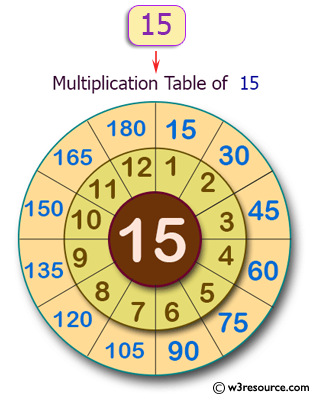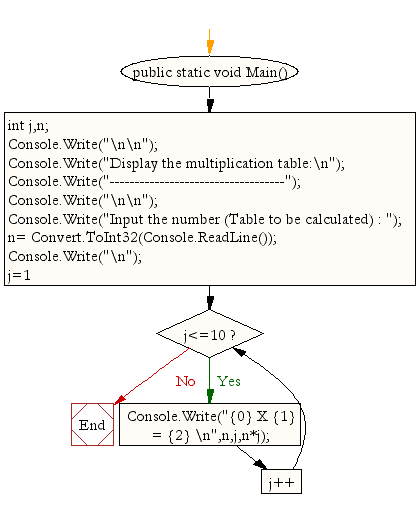﻿ C# - Compute multiplication table of a given integer# C# Sharp Exercises: Compute multiplication table of a given integer

## C# Sharp For Loop: Exercise-6 with Solution

Write a program in C# Sharp to display the multiplication table of a given integer.

Pictorial Presentation:Sample Solution:

C# Sharp Code:

``````using System;
public class Exercise6
{
public static void Main()
{
int j,n;

Console.Write("\n\n");
Console.Write("Display the multiplication table:\n");
Console.Write("-----------------------------------");
Console.Write("\n\n");

Console.Write("Input the number (Table to be calculated) : ");
Console.Write("\n");
for(j=1;j<=10;j++)
{
Console.Write("{0} X {1} = {2} \n",n,j,n*j);
}
}
}
```
```

Sample Output:

```Display the multiplication table:
-----------------------------------

Input the number (Table to be calculated) : 15

15 X 1 = 15
15 X 2 = 30
15 X 3 = 45
15 X 4 = 60
15 X 5 = 75
15 X 6 = 90
15 X 7 = 105
15 X 8 = 120
15 X 9 = 135
15 X 10 = 150
```

Flowchart:C# Sharp Code Editor:

Contribute your code and comments through Disqus.

What is the difficulty level of this exercise?

Test your Programming skills with w3resource's quiz.

﻿